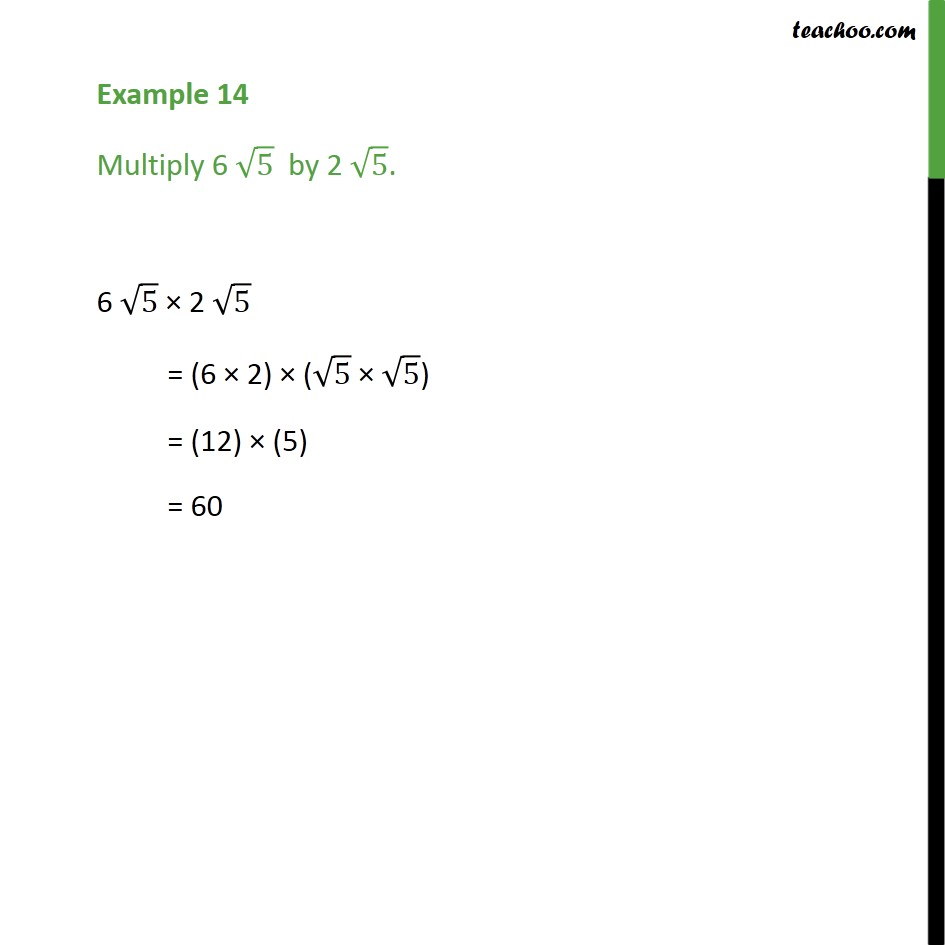Examples

Chapter 1 Class 9 Number Systems
Serial order wiseLearn in your speed, with individual attention - Teachoo Maths 1-on-1 Class

### Transcript

Example 13 Multiply 6 5 by 2 5. To multiply both root numbers We multiply whole numbers separately, and roots separately So, 6 5 2 5 = (6 2) ( 5 5) Here root 5 x root 5 becomes 5, = (12) (5) = 60 So, our multiplication is 60